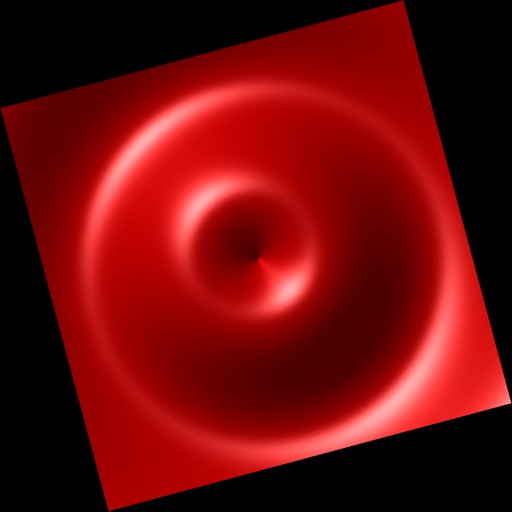## A LITTLE CALCULUS is a collection of more than 70 interactive topics from a first year calculus course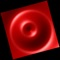# A Little Calculus

by David Little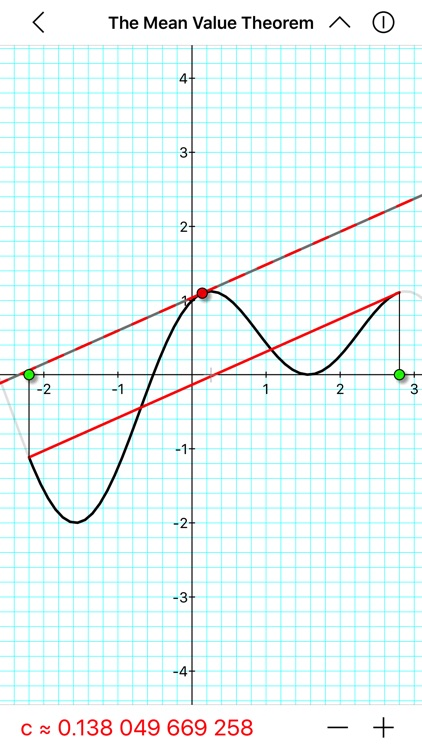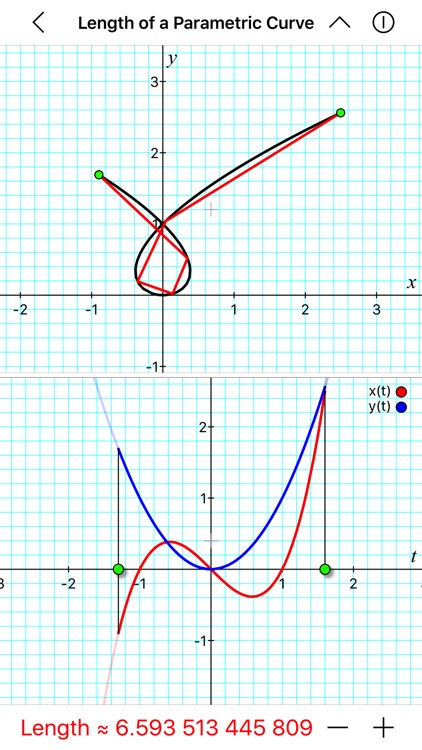A LITTLE CALCULUS is a collection of more than 70 interactive topics from a first year calculus course. To be used by both teachers and students, this app is designed to aid in the teaching and learning of calculus and should serve as a nice supplement to any introductory calculus textbook. While this app cannot be used to obtain solutions to calculus problems, it can be used to numerically and/or graphically verify computations for a wide variety of problems. Ultimately, the purpose of this app is to interact with and to gain a conceptual understanding of the fundamental topics of calculus.### App Details

Version
1.31
Rating
(11)
Size
41Mb
Genre
Education
Last updated
December 14, 2017
Release date
April 10, 2013

### App Screenshots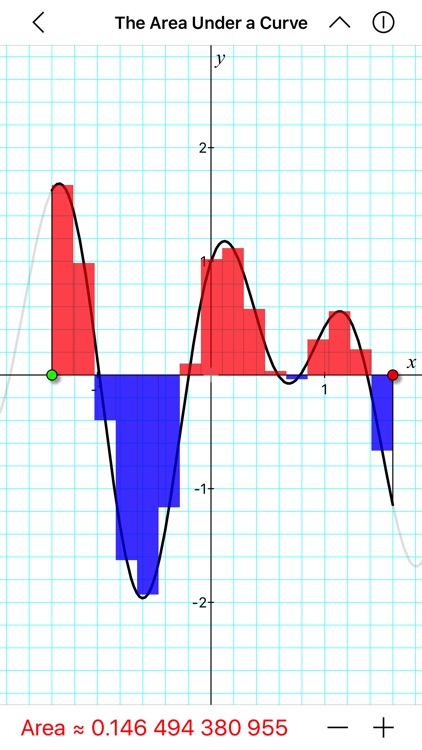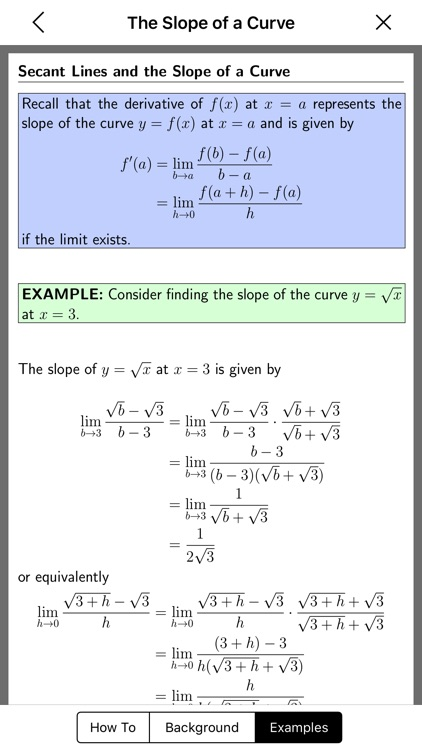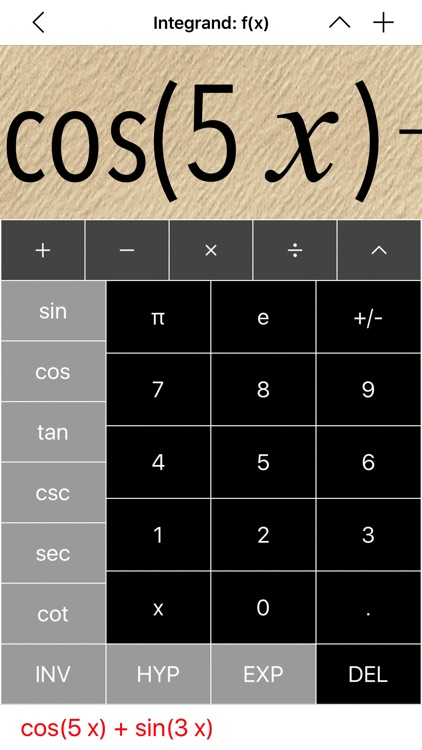### App Store Description

A LITTLE CALCULUS is a collection of more than 70 interactive topics from a first year calculus course. To be used by both teachers and students, this app is designed to aid in the teaching and learning of calculus and should serve as a nice supplement to any introductory calculus textbook. While this app cannot be used to obtain solutions to calculus problems, it can be used to numerically and/or graphically verify computations for a wide variety of problems. Ultimately, the purpose of this app is to interact with and to gain a conceptual understanding of the fundamental topics of calculus.

TOPICS:
• Graph functions of one variable, as well as parametric and polar curves.
• Investigate precalculus topics such as the equation of a line, graphing polynomials, Pythagorean Theorem, trigonometric functions, etc.
• Understand the fundamental notion of a limit from a formal or informal point of view.
• Study rates of change using the ideas from differential calculus (e.g., secant and tangent lines, derivatives, Mean Value Theorem, Newton's method, etc.)
• Calculate the area of a region or surface, length of a curve, and volume of a solid using the techniques of integral calculus.
• Interact with parametric and polar curves (e.g., slopes, areas, arc lengths, etc.)
• Learn about sequences and series (geometric, harmonic, alternating, etc.) and approximate functions using Taylor polynomials.
• Future releases to include more features, topics, and background information on each topic.

FEATURES:
• Customize each topic by changing any function, variable, and/or interval and by selecting from a variety of options.
• Interact with every graph (drag to change the value of a variable or to move/rotate the graph, pinch to zoom, etc.)
• Universal App (requires iOS 8.0 or later running on iPad, iPhone, or iPod Touch.)
• Fully functional, built-in calculator.